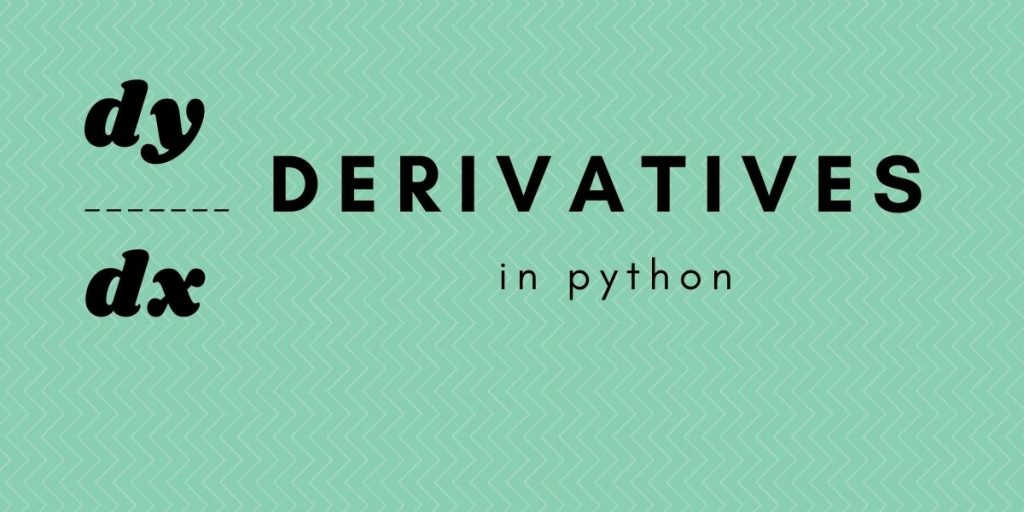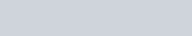# Derivatives in Python using SymPyHow to calculate derivatives in Python? In this article, we’ll use the Python SymPy library to play around with derivatives.

## What are derivatives?

Derivatives are the fundamental tools of Calculus. It is very useful for optimizing a loss function with gradient descent in Machine Learning is possible only because of derivatives.

Suppose we have a function y = f(x) which is dependent on x then the derivation of this function means the rate at which the value y of the function changes with the change in x.

This is by no means an article about the fundamentals of derivatives, it can’t be. Calculus is a different beast that requires special attention. I presume you have some background in calculus. This article is intended to demonstrate how we can differentiate a function using the Sympy library.

## Solving Derivatives in Python using SymPy

Python SymPy library is created for symbolic mathematics. The SymPy project aims to become a full-featured computer algebra system (CAS) while keeping the code simple to understand. Let’s see how to calculate derivatives in Python using SymPy.

### 1. Install SymPy using PIP

SymPy has more uses than just calculating derivatives but as of now, we’ll focus on derivatives. Let’s use PIP to install SymPy module.

```# pip install sympy
```

### 2. Solving a differential with SymPy diff()

For differentiation, SymPy provides us with the `diff` method to output the derivative of the function.

• Suppose we have a function: f(x) = x²
• Derivative of the function w.r.t x : f'(x) = 2x

Let’s see how can we achieve this using SymPy diff() function.

```#Importing sympy

from sympy import *

# create a "symbol" called x
x = Symbol('x')

#Define function
f = x**2

#Calculating Derivative
derivative_f = f.diff(x)

derivative_f
```

Declaring a symbol is similar to saying that our function has a variable ‘x’ or simply the function depends on x.

### 3. Solving Derivatives in Python

SymPy has `lambdify` function to calculate the derivative of the function that accepts symbol and the function as argument. Let’s look at example of calculating derivative using SymPy lambdify function.

```from sympy import *

# create a "symbol" called x
x = Symbol('x')

#Define function
f = x**2

f1 = lambdify(x, f)
#passing x=2 to the function
f1(2)
```

OUTPUT : 4

## Basic Derivative Rules in Python SymPy

There are certain rules we can use to calculate the derivative of differentiable functions.

Some of the most encountered rules are:

• Power Rule
• Product Rule
• Chain Rule
• Quotient Rule

Let’s dive into how can we actually use sympy to calculate derivatives as implied by the general differentiation rules.

### 1. Power Rule

In general : f'(xn) = nx(n-1)

Example, Function we have : f(x) = x⁵

It’s derivative will be : f'(x) = 5x(5-1) = 5x4

```import sympy as sym

#Power rule
x = sym.Symbol('x')
f = x**5
derivative_f = f.diff(x)
derivative_f
```

### 2. Product Rule

Let u(x) and v(x) be differentiable functions. Then the product of the functions u(x)v(x) is also differentiable.

``` (uv)′ = u′v + uv′
```

Example: f(x) = exp(x)*cos(x)

```import sympy as sym
#Product Rule
x = sym.Symbol('x')
f = sym.exp(x)*sym.cos(x)
derivative_f = f.diff(x)
derivative_f
```

### 3. Chain Rule

The chain rule calculate the derivative of a composition of functions.

• Say, we have a function h(x) = f( g(x) )
• Then according to chain rule: h′(x) = f ′(g(x)) g′(x)
• Example: f(x) = cos(x**2)

This process can be extended for quotient rule also. It must be obvious by now that only the function is changing while the application process remains the same, the remaining is taken care of by the library itself.

```import sympy as sym
#Chain Rule
x = sym.Symbol('x')
f = sym.cos(x**2)
derivative_f = f.diff(x)
derivative_f
```

## Python Partial Derivative using SymPy

The examples we saw above just had one variable. But we are more likely to encounter functions having more than one variables. Such derivatives are generally referred to as partial derivative.

A partial derivative of a multivariable function is a derivative with respect to one variable with all other variables held constant.

Example: f(x,y) = x4 + x * y4

Let’s partially differentiate the above derivatives in Python w.r.t x.

```import sympy as sym

#Derivatives of multivariable function

x , y = sym.symbols('x y')
f = x**4+x*y**4

#Differentiating partially w.r.t x
derivative_f = f.diff(x)
derivative_f
```

We use `symbols` method when the number of variables is more than 1. Now, differentiate the derivatives in Python partially w.r.t y

```import sympy as sym

#Derivatives of multivariable function

x , y = sym.symbols('x y')
f = x**4+x*y**4

#Differentiating partially w.r.t y
derivative_f = f.diff(y)
derivative_f
```

The code is exactly similar but now y is passed as input argument in `diff` method.

We can choose to partially differentiate function first w.r.t x and then y.

```import sympy as sym

#Derivatives of multivariable function

x , y = sym.symbols('x y')
f = x**4+x*y**4

#Differentiating partially w.r.t x and y
derivative_f = f.diff(x,y)
derivative_f
```Partial Differentiation w.r.t X And Y

## Summary

This article by no means was a course about derivatives or how can we solve derivatives in Python but an article about how can we leverage python SymPy packages to perform differentiation on functions. Derivatives are awesome and you should definitely get the idea behind it as they play a crucial role in Machine learning and beyond.